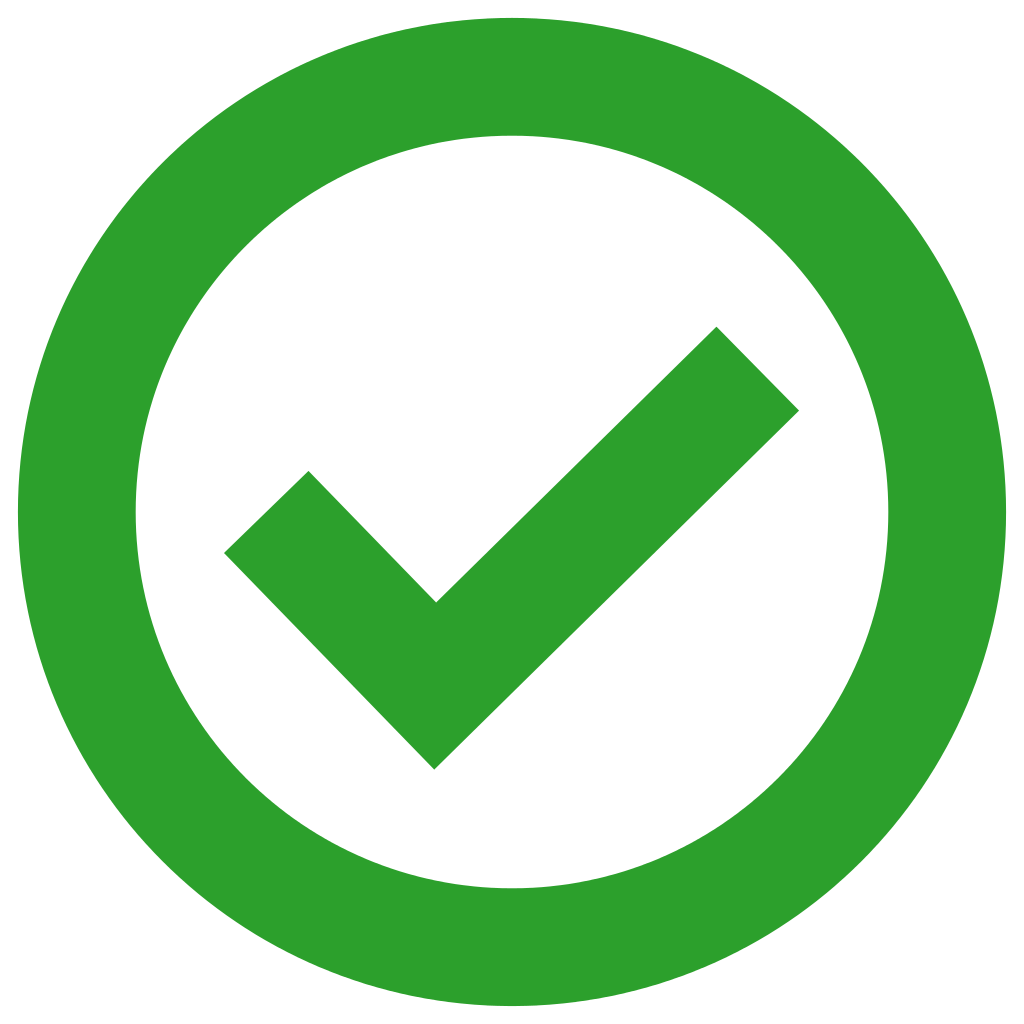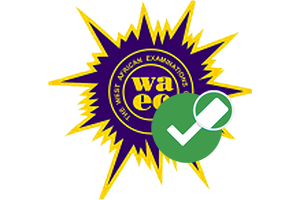###ONLINE CANDIDATES : 49### Confirmation Page# 2019/2020 WAEC GCE CHEMISTRY PRACTICAL EXPO QUESTIONS AND ANSWERS(2ai)
TEST: E + H2O(stirred)
INFERENCE: E is a mixture of salts

(2aii)
TEST: Residue
INFERENCE: Gas evolved is CO2 from HCO3- or CO3²-

(2aiii)
TEST: residue + HNO3 + H2O
INFERENCE : CO2 gas is evolved from HCO3-or CO3²-

(2aiv)
OBSERVATION: Blue gelatinous ppt soluble in drops. Soluble in excess to give deep blue ppt.
INFERENCE: Cu2+ present
Cu2+ confirmed

(2bi)
INFERENCE : Zn²+, pb²+, Al³+ present
Zn²+, pb²+ present

(2bii)
OBSERVATION: White gelatinous ppt soluble in excess Nh3 solution
INFERENCE: Zn²+, pb²+, Al³+
Zn²+ confirmed

(2biii)
TEST: Portion of filtrate of Ba(NO3)2 + HCL
INFERENCE: SO4²- or CO3²- present.
SO4²- confirmed.

(1a)
VA=11.30cm³
CA=5.3gldm³
VB=25.0cm³
CB=Ygldm³
HCl(aq)+NaOH(aq)—–>NaCl(aq)+H²O(i)
For acid NA; NB =1;1

(1ai)
Conc of A in moldm-³
=conc of A in gldm-³/molar mass in glmol
=5.3/36.5
HCl=>1+35.5=36.5glmole
=0.145moldm-³

CAVA/CBVB=NA/NB
0.145*11.30/CB*25.0=1/1
1.6385=25CB
CB=1.6385/25
CB=0.06554moldm³

(1aii)
Conc of B in moldm-³=
Conc of A in gldm-³/molar mass in glmol

Molar mass of NaOH
=Na+O+ H
=23+16+1
=40glmol .
0.066=y/40
y=0.066*40
y=2.64

(1aiii)
HCl+NaOH—–>NaCl+H²O
1mole of HCl give 1mole NaCl

1.6385mole
n=CV
=0.145*11.30
=1.6385mole
No of mole =mass/molar mass
1.6385=mass/58.5
Molar mass of NaCl=58.5glmole
Mass of NaCl =58.5*16.385
=95.85g

(1bi)
The indicator used is methyl orange because the titration is between the strong acid and strong base

(1bii)
Orange color would be shown at the end point .

(3a)
-Glucose-
The most important monosaccharide is glucose, the body’s preferred energy source. Glucose is also called blood sugar, as it circulates in the blood, and relies on the enzymes glucokinase or hexokinase to initiate metabolism.

-Sucrose-
Sucrose is commonly known as table sugar, and is obtained from sugar cane or sugar beets. Fruits and vegetables also naturally contain sucrose.

CHECK OUT:  2019/2020 WAEC GCE GEOGRAPHY PRACTICAL ANSWER

(3bi)
(i)potassium iodide
(ii)throsulphate

(3bii)
Starch indicator

(3biii)
(i)before end point —-> dark color
(ii)at the end point —-> colourless

(3c)
(i)soda lime is preferred because it does not attack rubber nor dry.
(ii)it is not deliquescentJoin Our Telegram Channel |Whatsapp Our Admins: Click Any Name Mr.Paul or Mr.Henry

### Comment

Posted by on 11th September 2019.

Categories: Waec Gce